## What is the Circumference of a Circle and How to Measure it?

Circumference Calculator

#### What is the circumference of a circle?

The perimeter of a circle is known as its circumference.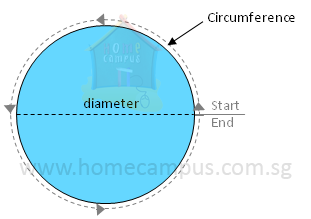Trace the outline of a few circular objects of different sizes like a CD, a circular bottle lid, a frisbee, a round bowl and a key ring on paper and cut them along their circumference. Then, do the following:
1. Measure the diameter of each circle by folding in half.
2. Measure the circumference of each circle using a string.
3. Record both readings in a table as below.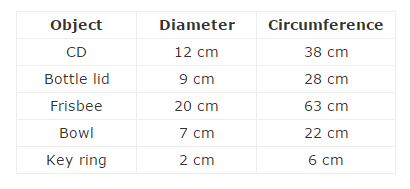As you will notice, the circumference of each object is about 3 times its diameter.
More precisely, the circumference of a circle is
 22 7
times its diameter.
The value
 22 7
is represented by π and read as pi.
The approximate value of π is 3.14.

#### What is the formula to calculate circumference of a circle?

Circumference  =  π × Diameter
Or,
Circumference  =  π  ×  2 × Radius  =  2  ×  π  ×  Radius
Practice Unlimited Circumference Questions

#### 1. Find the circumference of the circles below.

a)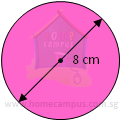Diameter  =  8 cm
Circumference  =  π  ×  8 cm  =  3.14  ×  8 cm  =  25.12 cm
b)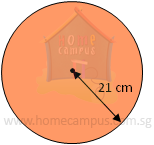Circumference
=  2  ×  π  ×  21 cm
=  2  ×
 22 17
×  213 cm
132 cm
When calculation is easy, we use
 22 7
instead of 3.14 as the value of π

#### 2. Find the perimeters of the following figures. Round off your answers to 2 decimal places.

a)   A semicircle of diameter 17.6 cm.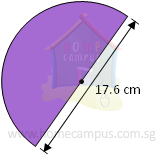Perimeter of a semicircle
=  Half the circumference of the circle  +  diameter
=  (
 1 2
×  π  ×  17.6)  +  17.6
=  (
 1 2
×  3.14  ×  17.6)  +  17.6
=  27.632  +  17.6
=  45.232
≈  45.23 cm=  A quarter of the circumference of circle  +  (2  ×  radius)
=  (
 1 24
×  21  ×  3.14  ×  5)  +  (2  ×  5)
=  7.85  +  10
=  17.85 cm
c)   A three-quarter circle of radius 84 cm.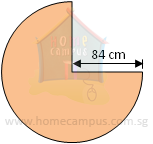Perimeter of
 3 4
circle
 3 4
of the circumference of the circle  +  (2  ×  radius)
=  (
 3 24
×  21  ×
 22 17
×  8412)  +  (2  ×  84)
=  (
 3 12
×  1  ×
 2211 1
×  12)  +  168
=  396  +  168
=  564 cm

#### 3. Can a pizza of radius 12 cm fit into a plate that has a circumference of 100 cm?

Circumference of pizza  =  2  ×  3.14  ×  12  =  75.36 cm

75.36 cm  <  100 cm

Yes, the pizza can fit into the plate.

#### 4. The spoke of a wheel is 35 cm long. How far does the wheel travel in 1 rotation? Give your answer in metres.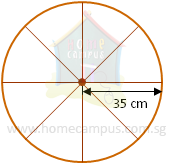Spoke of the wheel  =  Radius of the wheel

1 rotation of the wheel
=  Circumference of the wheel
=  2  ×
 22 17
×  355 cm
=  220 cm
=  2.2 m

The wheel travels 2.2 m in 1 rotation.